Math - 2017 Past Year Paper

# Math - 2017 Past Year Paper - IIT JAM

Test Description

## 60 Questions MCQ Test IIT JAM Past Year Papers and Model Test Paper (All Branches) - Math - 2017 Past Year Paper

Math - 2017 Past Year Paper for IIT JAM 2023 is part of IIT JAM Past Year Papers and Model Test Paper (All Branches) preparation. The Math - 2017 Past Year Paper questions and answers have been prepared according to the IIT JAM exam syllabus.The Math - 2017 Past Year Paper MCQs are made for IIT JAM 2023 Exam. Find important definitions, questions, notes, meanings, examples, exercises, MCQs and online tests for Math - 2017 Past Year Paper below.
Solutions of Math - 2017 Past Year Paper questions in English are available as part of our IIT JAM Past Year Papers and Model Test Paper (All Branches) for IIT JAM & Math - 2017 Past Year Paper solutions in Hindi for IIT JAM Past Year Papers and Model Test Paper (All Branches) course. Download more important topics, notes, lectures and mock test series for IIT JAM Exam by signing up for free. Attempt Math - 2017 Past Year Paper | 60 questions in 180 minutes | Mock test for IIT JAM preparation | Free important questions MCQ to study IIT JAM Past Year Papers and Model Test Paper (All Branches) for IIT JAM Exam | Download free PDF with solutions
 1 Crore+ students have signed up on EduRev. Have you?
Math - 2017 Past Year Paper - Question 1

### Consider the function f(x, y) = 5 – 4 sin x + y2 for 0 < x < 2p and y ∈ R. The set of critical points of f(x, y) consists of

Math - 2017 Past Year Paper - Question 2

### Let φ : R → R be a differentiable function such that φ' is strictly increasing with φ(1) = 0. Let a and b denote the minimum and maximum values of φ(x) on the interval [2, 3], respectively. Then which one of the following is TRUE ?

Math - 2017 Past Year Paper - Question 3

### The number of generators of the additive group Z36 is equal to

Math - 2017 Past Year Paper - Question 4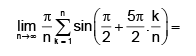Math - 2017 Past Year Paper - Question 5

Let f : R → R be a twice differentiable function. If g(u, v) = f(u2 – v2), then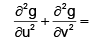Math - 2017 Past Year Paper - Question 6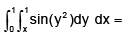Math - 2017 Past Year Paper - Question 7

Let f1(x), f2(x), g1(x), g2(x) be differentiable functions on R.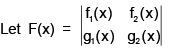be the determinent of the matrix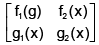. Then F'(x) is equal to

Math - 2017 Past Year Paper - Question 8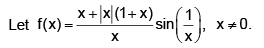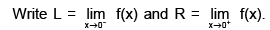Then which one of the following is TRUE ?

Math - 2017 Past Year Paper - Question 9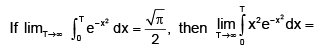Math - 2017 Past Year Paper - Question 10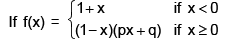satisfies the assumptions of Rolle’s theorem in the interval [–1, 1], then the ordered pair (p, q) is

Math - 2017 Past Year Paper - Question 11

The flux of the vector field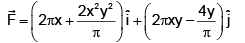along the outward normal, across the ellipse x2 + 16y2 = 4 is equal to

Math - 2017 Past Year Paper - Question 12

Let M be the set of all invertible 5 × 5 matrices with entries 0 and 1. For each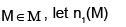and n0(M) denote the number of 1’s and 0’s in M, respectively. Then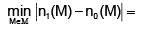Math - 2017 Past Year Paper - Question 13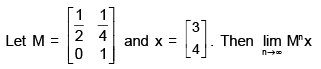Math - 2017 Past Year Paper - Question 14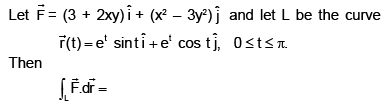Math - 2017 Past Year Paper - Question 15

The line integral of the vector field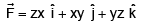along the boundary of the triangle with vertices (1,0,0), (0,1,0) and (0,0,1), oriented anticlockwise, when viewed from the point (2,2,2) is

Math - 2017 Past Year Paper - Question 16

The area of the surface z = xy/3 intercepted by the cylinder x2 + y2  ≤ 16 lies in the interval

Math - 2017 Past Year Paper - Question 17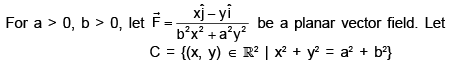be the circle oriented anti- clockwise. Then

Math - 2017 Past Year Paper - Question 18

The flux of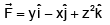along the outward normal, across the surface of the solid is equal to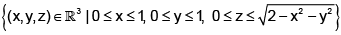Math - 2017 Past Year Paper - Question 19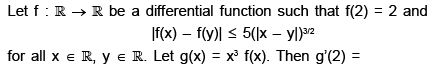Math - 2017 Past Year Paper - Question 20

Let f : R → [0, ∞) be a continuous function. Then which one of the following is NOT TRUE ?

Math - 2017 Past Year Paper - Question 21

The interval of convergence of the power series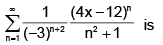Math - 2017 Past Year Paper - Question 22

Let P3 denote the real vector space of all polynomials with real coefficients of degree at most 3. Consider the map T : P→ P3 given by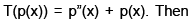Math - 2017 Past Year Paper - Question 23

Let f(x, y) =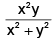for (x, y) ≠ (0, 0). Then

Math - 2017 Past Year Paper - Question 24

Let S be an infinite subset of R such that S\{a} is compact for some α ∈ S. Then which one of the following is TRUE ?

Math - 2017 Past Year Paper - Question 25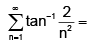Math - 2017 Past Year Paper - Question 26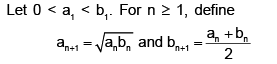Then which one of the followings is NOT TRUE ?

Math - 2017 Past Year Paper - Question 27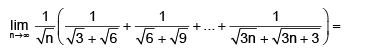Math - 2017 Past Year Paper - Question 28

Which one of the following is TRUE ?

Math - 2017 Past Year Paper - Question 29

A particular integral of the differential equation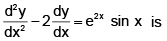Math - 2017 Past Year Paper - Question 30

Let y(x) be the solution of the differential equation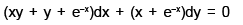satisfying y(0) = 1. Then y(–1) is equal to

*Multiple options can be correct
Math - 2017 Past Year Paper - Question 31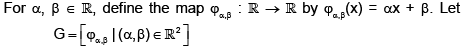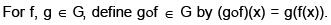Then which of the following statements is/are TRUE ?

*Multiple options can be correct
Math - 2017 Past Year Paper - Question 32

The volume of the solid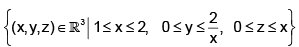is expressible as

*Multiple options can be correct
Math - 2017 Past Year Paper - Question 33

Let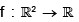be a function. Then which of the following statements is/are TRUE ?

*Multiple options can be correct
Math - 2017 Past Year Paper - Question 34

If X and Y are n × n matrices with real entries, then which of the following is/are TRUE ?

*Multiple options can be correct
Math - 2017 Past Year Paper - Question 35

Let G be a group of order 20 in which the conjugacy classes have sizes 1, 4, 5, 5, 5. Then which of the followings is/are TRUE ?

*Multiple options can be correct
Math - 2017 Past Year Paper - Question 36

Let {xn} be a real sequence such that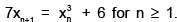Then which of the following statements is/are TRUE ?

*Multiple options can be correct
Math - 2017 Past Year Paper - Question 37

Let S be the set of all rational numbers in (0, 1). Then which of the following statements is/are TRUE ?

*Multiple options can be correct
Math - 2017 Past Year Paper - Question 38

Let M be an n × n matrix with real entries such that M3 = I. Suppose that Mv ≠ v for any nonzero vector v. Then which of the following statements is/are TRUE ?

*Multiple options can be correct
Math - 2017 Past Year Paper - Question 39

Let y(x) be the solution of the differential equation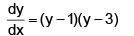satisfying the condition y(0) = 2. Then which of the following is/are TRUE ?

*Multiple options can be correct
Math - 2017 Past Year Paper - Question 40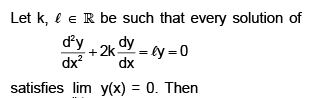*Answer can only contain numeric values
Math - 2017 Past Year Paper - Question 41

Evaluation of 83 × 82 × 8-5 is.......

*Answer can only contain numeric values
Math - 2017 Past Year Paper - Question 42

Let G be a subgroup of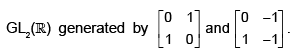Then the order of G is -----

*Answer can only contain numeric values
Math - 2017 Past Year Paper - Question 43

Consider the permutations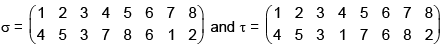in S8 . The number of η ∈  S8  such that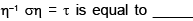*Answer can only contain numeric values
Math - 2017 Past Year Paper - Question 44

Let P be the point on the surface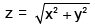closet to the point (4,2,0). Then the square of the distance between the origin and P is ______.

*Answer can only contain numeric values
Math - 2017 Past Year Paper - Question 45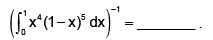*Answer can only contain numeric values
Math - 2017 Past Year Paper - Question 46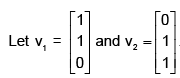Let M be the matrix whose columns are v1, v2, 2v1 – v2, v1 + 2v2 in that order. Then the number of linearly independent solutions of the homogeneous system of linear equations Mx = 0 is __________.

*Answer can only contain numeric values
Math - 2017 Past Year Paper - Question 47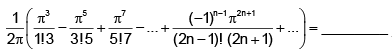*Answer can only contain numeric values
Math - 2017 Past Year Paper - Question 48

Let P be a 7 × 7 matrix of rank 4 with real entries. Let a ∈ R7 be a column vector. Then the rank of P + aaT is at least ________.

*Answer can only contain numeric values
Math - 2017 Past Year Paper - Question 49

For x > 0, let |x| denote the greatest integer less than or equal to x. Then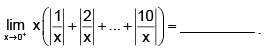Detailed Solution for Math - 2017 Past Year Paper - Question 49

Solution :-  lim x -->0  1/x  => 1/0

= ∞ i.e Apply L-hospital rule

lim x-->0 x[1/x + 2/x + 3/x + 4/x +.......................10/x]

lim x→0  x[10*11]/2 * 1/x

= [10*11]/2    => 55

*Answer can only contain numeric values
Math - 2017 Past Year Paper - Question 50

The number of subgroups of Z7 x Zof order 7 is _______.

*Answer can only contain numeric values
Math - 2017 Past Year Paper - Question 51

Let y(x), x > 0 be the solution of the differential equation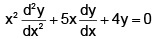satisfying the conditions y(1) = 1 and y’(1) = 0. Then the value of e2y(e) is ______

*Answer can only contain numeric values
Math - 2017 Past Year Paper - Question 52

Let T be the smallest positive real number such that the tangent to the helix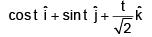at t = T is orthogonal to the tangent at t = 0. Then the line integral of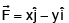along the section of the helix from t = 0 to t = T is _____

*Answer can only contain numeric values
Math - 2017 Past Year Paper - Question 53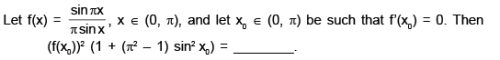*Answer can only contain numeric values
Math - 2017 Past Year Paper - Question 54

The maximum order of a permutation s in the symmetric group S10 is ____

*Answer can only contain numeric values
Math - 2017 Past Year Paper - Question 55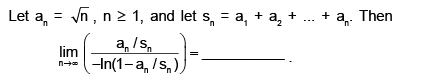*Answer can only contain numeric values
Math - 2017 Past Year Paper - Question 56

For a real number x, define [x] to be the smallest integer greater than or equal to x. Then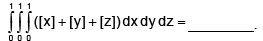*Answer can only contain numeric values
Math - 2017 Past Year Paper - Question 57

For x > 1, let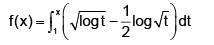The number of tangents to the curve y = f(x) parallel to the line x + y = 0 is ____

*Answer can only contain numeric values
Math - 2017 Past Year Paper - Question 58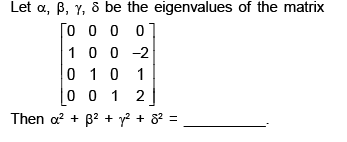*Answer can only contain numeric values
Math - 2017 Past Year Paper - Question 59

The radius of convergence of the power series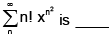*Answer can only contain numeric values
Math - 2017 Past Year Paper - Question 60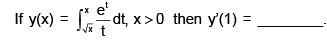## IIT JAM Past Year Papers and Model Test Paper (All Branches)

8 docs|48 tests
Information about Math - 2017 Past Year Paper Page
In this test you can find the Exam questions for Math - 2017 Past Year Paper solved & explained in the simplest way possible. Besides giving Questions and answers for Math - 2017 Past Year Paper, EduRev gives you an ample number of Online tests for practice

## IIT JAM Past Year Papers and Model Test Paper (All Branches)

8 docs|48 tests(Scan QR code)